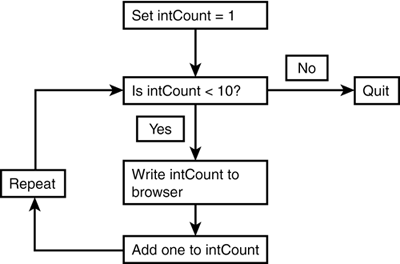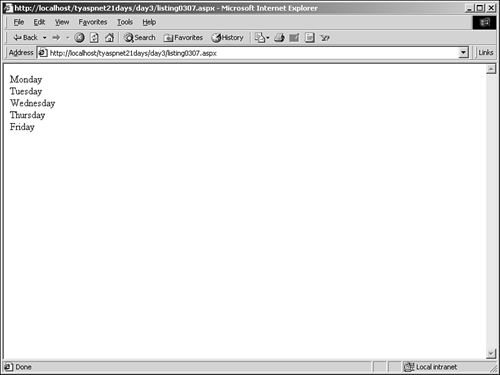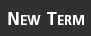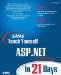# Looping Logic

 IOTA^_^Sams Teach Yourself ASP.NET in 21 Days, Second EditionBy Chris Payne Table of Contents Day 3.  Using Visual Basic.NET and C#

Loops allow you to perform an action over and over again until some condition is met. There are three types of loops in VB.NET and C#: While, Do, and For.

#### While Loops

While loops are useful when you know don't know how many times you need to do something, such as when you want to loop through an unknown number of cars until you run into a Mercedes. These loops are based on conditional expressions, and they continue to run until the condition becomes false. The syntax for a while loop in VB.NET is as follows:

` While condition    code End While `

In C#:

` while (condition) {    code } `

For example, the following code counts from 1 to 10 and prints out the number to the browser:

` int intCount = 1; while (intCount < 10) {    Response.Write(intCount + "<br>");    intCount += 1; } `

On the second line, the program checks if intCount is greater than 10. It shouldn't be, because you just set it to be equal to 1. The program executes the code inside the while loop, writing the number to the browser and adding 1 to intCount. It then goes back to the second line and checks if intCount is now greater than 10. intCount is 2 now, so the loop continues. When it finishes, you'll see the numbers 1 through 9 displayed in the browser. Figure 3.5 illustrates this process.

##### Figure 3.5. The looping process.Why do we use 9 instead of 10? Once intCount hits 10, the statement on line 2 is no longer true it evaluates to false so the loop exits and continues after line 5.

The do loop is the same as the while loop; the syntax is just slightly different. The do loop comes in many forms, such as the following:

` 'VB.NET Do    code Loop While condition //C# do {    code } while condition `

Here's another one:

` Do While condition    code Loop `

The different forms produce different output depending on how many times you want them to execute. Let's look at an example of each:

` dim intCount as Integer = 10 Do    Response.Write(intCount & "<br>")    intCount = intCount + 1 Loop While intCount < 10 Do While intCount < 10    Response.Write(intCount & "<br>")    intCount = intCount + 1 Loop `

The first loop prints out the number 10, while the second loop prints out nothing. Why? The first loop evaluates the condition on the last line of the loop. Therefore, the code inside the loop will be executed once no matter what. The second loop evaluates the condition right up front, and in this case it stops the code from ever executing.

You should use a Do loop when you want the code inside the block to execute at least once. Conversely, you should use a Do While loop if you don't care whether the code is executed at all. In VB.NET, you can also use until in place of while:

` dim intCount as Integer = 1 Do    Response.Write(intCount & "<br>")    intCount = intCount + 1 Loop Until intCount >= 10 `

This loop does exactly the same thing as the first loop in the previous listing. The difference is that using until will let the loop go until the condition is true, whereas using while stops when the condition is false. In this case, the loop stops when intCount is greater than or equal to 10.

#### For Loops

Use a for loop when you know how many times you want to execute your code. This loop increments a counter to tell itself when to stop executing. The for loop syntax is as follows:

` 'VB.NET For variable = startvalue To stopvalue [Step step-size]    code Next [variable] //C# for (variable = startvalue; variable comparison stopvalue; step) {    code } `

This loop uses the step-size to increment variable until it reaches the stopvalue, at which point the loop stops executing. (In VB.NET, you can leave the step parameter out if you want it defaults to a value of 1.) For example:

` For intCount = 1 to 10    Response.Write(intCount & "<br>") Next `

or:

` For intCount = 10 to 1 step  1    Response.Write(intCount & "<br>") Next `

The first loop prints out the numbers 1 through 10, while the second prints them in reverse order.

In C#, the previous code snippet would be

` for (intCount = 10; intCount == 1; intCount--) {    Response.Write(intCount + "<br>"); } `

Another form of the for loop is the for...each loop (foreach in C#). This loop iterates through all of the items in a collection, such as an array. For example:

` dim arrWeekDays() as String = {"Monday", "Tuesday", _    "Wednesday", "Thursday", "Friday"} For Each strDay in arrWeekDays    Response.Write(strDay & "<br>") Next `

On the first line, you create an array of strings containing the weekdays. On the fourth line, you loop through each item in the array. The variable strDay is simply a counter that the loop uses you could specify any variable here. The loop assigns each variable in the array to strDay, and you print that value out on the fifth line. The result is that the days of the week are displayed in the browser, as shown in Figure 3.6.

##### Figure 3.6. Using a for...each loop to iterate over the items in an array.#### Infinite LoopsNotice that you had to manually increment the counter in your while and do loops, whereas the for loops did so automatically. It's very important to increment your counter in these loops. Otherwise, you'd end up with an infinite loop a loop that never exits. Not only is this a pain for visitors to your site, but it also drains system resources very quickly and can cause the site to crash.

If you ever need to exit a loop before it finishes, you can use the exit (break in C#) keyword to stop the loop. The syntax for this keyword in VB.NET depends on where you use it. For example:

` Do While intCount < 10    Response.Write(intCount & "<br>")    intCount = intCount + 1    if intCount = 7 then       exit do    end if Loop For intCount = 1 to 10    Response.Write(intCount & "<br>")    if intCount = 7 then       exit for    end if Next `

The first loop iterates through the numbers 1 through 10, but if the number equals 7 (which it eventually will, in this case), you stop the loop by using exit do (note that it prints out only 1 6). The second loop does exactly the same thing, but using exit for (this one prints out 1 7). The exit statement that's used will depend on the type of loop you're in.

In C#, the syntax doesn't change no matter where the keyword is used:

` for (intCount = 1; intCount == 10; intCount++) {    Response.Write(intCount + "<br>");    if (intCount == 7) {       break;    } } `

 IOTA^_^
 TopSams Teach Yourself ASP.NET in 21 Days (2nd Edition)
ISBN: 0672324458
EAN: 2147483647
Year: 2003
Pages: 307
Authors: Chris Payne

Similar book on Amazon